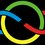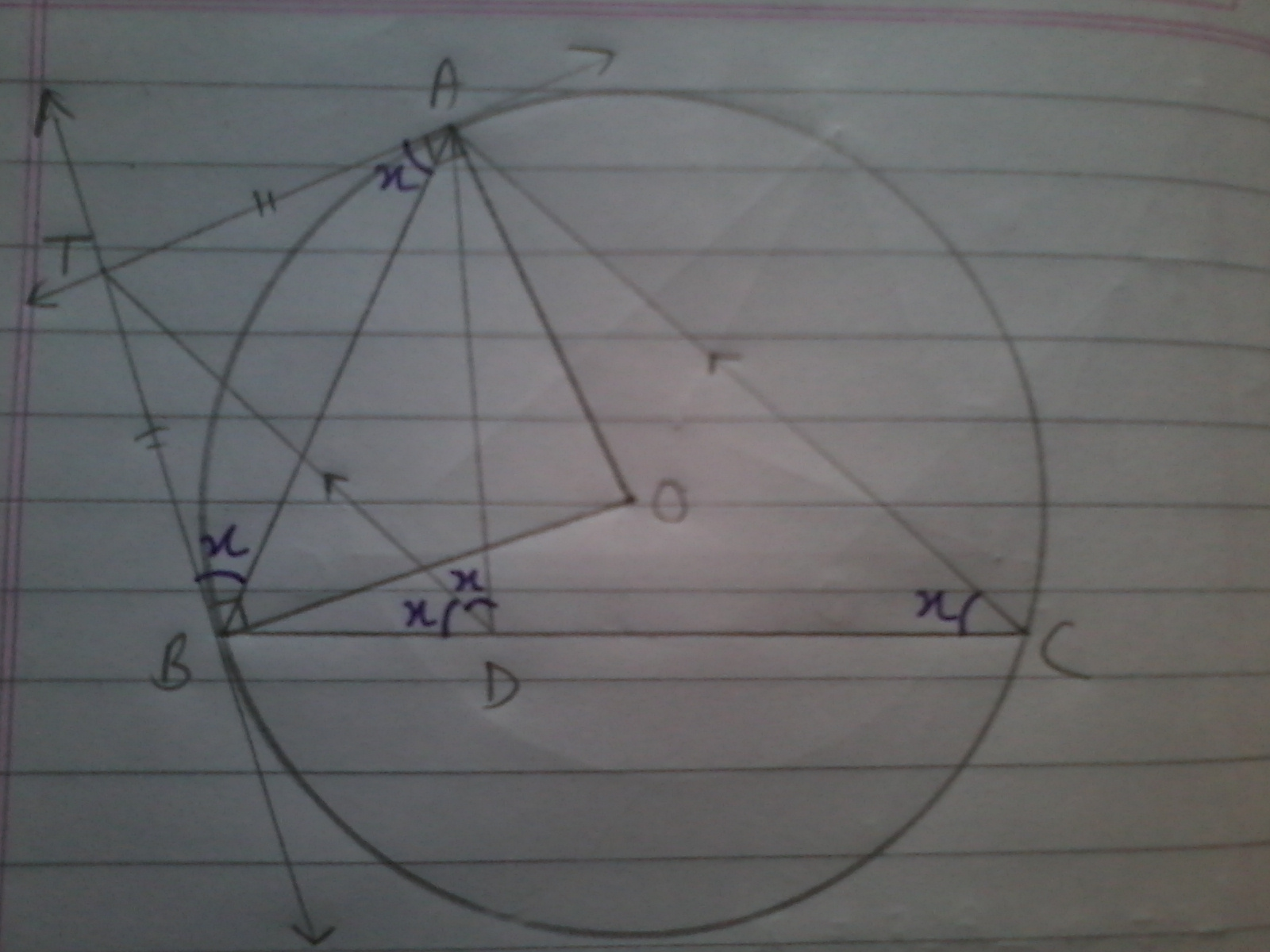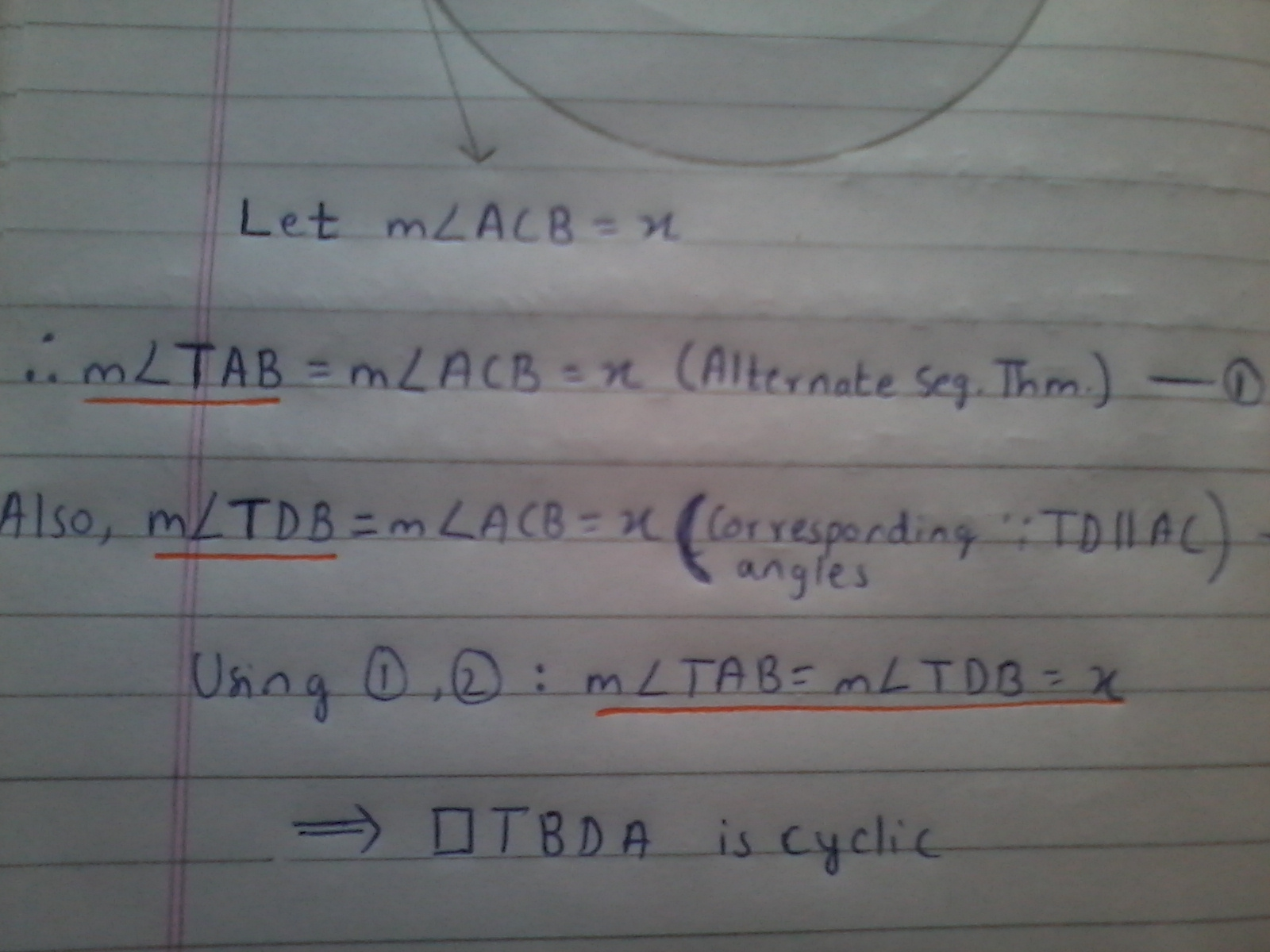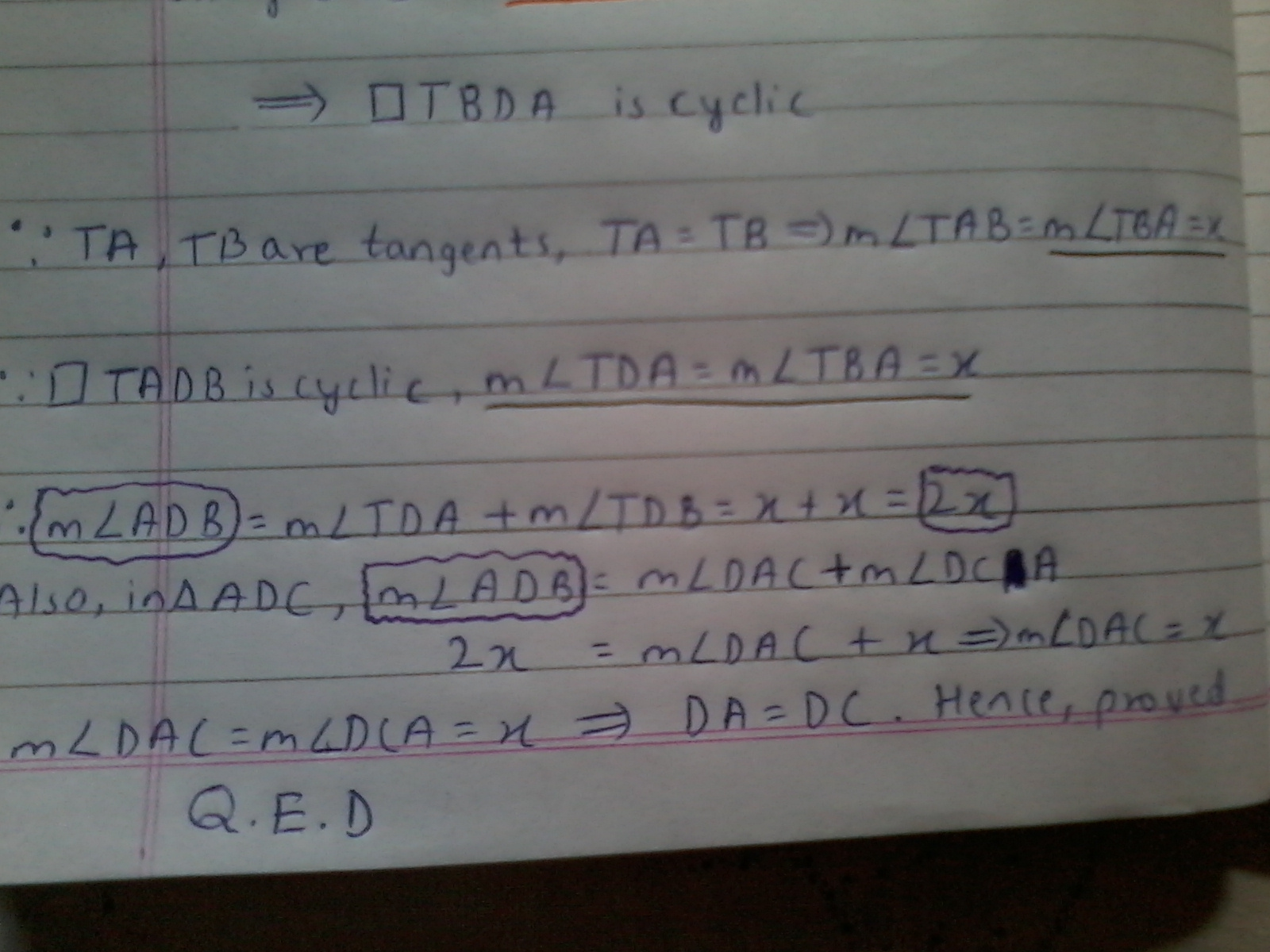# Synthetic Geometry Group-Sualeh's Proposal (Pakistan Round 1)

These problems are my first submissions to Xuming's Geometry group.

However they are for the purpose of sharing the Geometry problems from Pakistan's First Round (for 2016)

Q1.The tangents at $$A,B$$ to the circumcircle$$\omega$$ of Triangle $$ABC$$ meet at $$T$$. The line through $$T||AC$$ meets $$BC$$ at $$D$$. Prove that $$AD=CD$$.

Q2. In a right angled triangle $ABC, \hat{C}=90^{\circ}, CD\perp AB$ at $D$ and the angle bisector of $\hat{B}$ intersects $CD,AC$ at $O,E$ respectively. Through $O$ introduce $FG \parallel AB$ such that $FG$ intersects $AC,BC$ at $F,G$ respectively. Prove that $AF=CE$.

###### Geometry-Pakistan's First Round (for IMO 2016).Note by Sualeh Asif
5 years, 10 months ago

This discussion board is a place to discuss our Daily Challenges and the math and science related to those challenges. Explanations are more than just a solution — they should explain the steps and thinking strategies that you used to obtain the solution. Comments should further the discussion of math and science.

When posting on Brilliant:

• Use the emojis to react to an explanation, whether you're congratulating a job well done , or just really confused .
• Ask specific questions about the challenge or the steps in somebody's explanation. Well-posed questions can add a lot to the discussion, but posting "I don't understand!" doesn't help anyone.
• Try to contribute something new to the discussion, whether it is an extension, generalization or other idea related to the challenge.

MarkdownAppears as
*italics* or _italics_ italics
**bold** or __bold__ bold
- bulleted- list
• bulleted
• list
1. numbered2. list
1. numbered
2. list
Note: you must add a full line of space before and after lists for them to show up correctly
paragraph 1paragraph 2

paragraph 1

paragraph 2

[example link](https://brilliant.org)example link
> This is a quote
This is a quote
    # I indented these lines
# 4 spaces, and now they show
# up as a code block.

print "hello world"
# I indented these lines
# 4 spaces, and now they show
# up as a code block.

print "hello world"
MathAppears as
Remember to wrap math in $$ ... $$ or $ ... $ to ensure proper formatting.
2 \times 3 $2 \times 3$
2^{34} $2^{34}$
a_{i-1} $a_{i-1}$
\frac{2}{3} $\frac{2}{3}$
\sqrt{2} $\sqrt{2}$
\sum_{i=1}^3 $\sum_{i=1}^3$
\sin \theta $\sin \theta$
\boxed{123} $\boxed{123}$

Sort by:

Due to lack of time I have uploaded pics of my solution to problem 1.- 5 years, 10 months ago

Good job Nihar ! The crux move was to identify that TBDA is cyclic, by wishful thinking.

- 5 years, 10 months ago

absolutely!

- 5 years, 10 months ago

@Calvin Lin @Xuming Liang Here is my submission!

@Nihar Mahajan ,@Sharky Kesa I thought you would be interested!

- 5 years, 10 months ago

Ill add in the solutions when requested. (Not before a week though)

- 5 years, 10 months ago

1st one is just some simple angle chase using alternate segment theorem as proved in the solution by @Nihar Mahajan.

2nd one is also just trivial: Solution to II.

$\Delta DBC \sim \Delta CBA$

=> $\dfrac{DB}{CB} = \dfrac{CB}{AB}$

=> $\dfrac{DO}{OC} = \dfrac{CE}{AE}$ --- (Int. Angle Bisector Theorem)

=> $\dfrac{AF}{CF} = \dfrac{CE}{AE}$

=> $\dfrac{AF}{AC} = \dfrac{CE}{AC}$ ---- (By rule of Dividendo)

=> $AF = AC$

K.I.P.K.I.G

- 4 years, 3 months ago